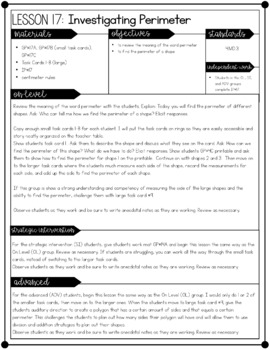# 4th Grade Guided Math - Measurement and DataSubject
Resource Type
Format
PDF (98 MB|239 pages)
Standards
\$15.00
Also included in
1. Fourth Grade Guided Math: This 4th grade Guided Math Yearlong Curriculum Bundle incorporates EVERYTHING you will need to easily and effectively implement Guided Math in your 4th Grade classroom. Implementing Guided Math in fourth grade has never been easier! Check out this Guided Math curriculum bun
\$96.00
\$120.00
Save \$24.00
• Product Description
• Standards

Fourth Grade Guided Math: Measurement and Data and Measurement and Data Lessons: This is the seventh unit in my 4th Grade Guided Math curriculum. This unit focuses on Measurement and Data and includes EVERYTHING you will need to quickly and efficiently implement Guided Math in your 4th Grade classroom.

It is completely aligned to the Common Core State Standards.

This 4th Grade Guided Math resource includes 21 DIFFERENTIATED lessons on the following concepts:

* Introduction to Capacity

* Measuring in Liters and Milliliters

* Problem Solving

* Customary Units of Capacity

* Capacity Conversions

* Introduction to Mass and Weight

* Measuring Mass in Kilograms and Grams

* Measuring Weight in Pounds and Ounces

* Introduction to Measurement

* Measuring with Meters

* Measuring Inches, Feet, and Yards

* Measuring and Recording Data on a Line Plot

* Problem Solving with Measurement

* Introduction to Area and Perimeter

* Investigating Perimeter

* Finding an Unknown Side Length

* Investigating Area

* Area and Perimeter of a Rectangle

* Area of Composite Figures

This 4th Grade Guided Math Measurement and Data Resource includes:

☞ Fully Detailed and Differentiated Lesson Plans for Small Group Instruction

☞ Information Guide on HOW TO effectively implement Guided

Math in your classroom (images and text instructions)

☞ Independent Practice Printables / Interactive Notebook activities

aligned to each small group instruction lesson

☞ Guided Practice Workmats for EACH lesson to help guide your

small group instruction

☞ 20 Word Problems for Math Journals

☞ 15 Math Stations - 5 SPIN-ITS Math Stations, 5 Roll-Its Math Stations, and 5 Solve-Its Math Stations

☞ Pre and Post Assessments on decimals

☞ Guided Math Organization tools - Guided Math Binder Cover,

Binder Spines, Binder dividers lesson plan templates, anecdotal

notes template, weekly lesson plan template, math journal labels,

math station labels, large poster-sized iChart (print at office

supply store), math station recording sheet, and fact fluency

station labels

☞ Links to resources and tools that I use during my Guided Math

instruction

This Guided Math Measurement and Data Resource is JAM-PACKED with EVERYTHING you need to quickly and efficiently implement Guided Math in your 4th Grade Classroom!

If you are interested in this resource, please check out the BUNDLE to save a BUNDLE!

to see state-specific standards (only available in the US).
Apply the area and perimeter formulas for rectangles in real world and mathematical problems. For example, find the width of a rectangular room given the area of the flooring and the length, by viewing the area formula as a multiplication equation with an unknown factor.
Use the four operations to solve word problems involving distances, intervals of time, liquid volumes, masses of objects, and money, including problems involving simple fractions or decimals, and problems that require expressing measurements given in a larger unit in terms of a smaller unit. Represent measurement quantities using diagrams such as number line diagrams that feature a measurement scale.
Know relative sizes of measurement units within one system of units including km, m, cm; kg, g; lb, oz.; l, ml; hr, min, sec. Within a single system of measurement, express measurements in a larger unit in terms of a smaller unit. Record measurement equivalents in a two-column table. For example, know that 1 ft is 12 times as long as 1 in. Express the length of a 4 ft snake as 48 in. Generate a conversion table for feet and inches listing the number pairs (1, 12), (2, 24), (3, 36),...
Total Pages
239 pages
N/A
Teaching Duration
1 month
Report this Resource to TpT
Reported resources will be reviewed by our team. Report this resource to let us know if this resource violates TpT’s content guidelines.
• Ratings & Reviews
• Q & A

Teachers Pay Teachers is an online marketplace where teachers buy and sell original educational materials.